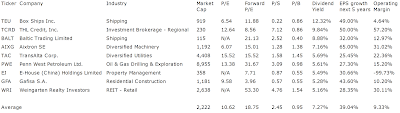## Pages

### 9 High Yield Stocks With Highest Earnings Growth

High Yielding Stocks With Fast Earnings Per Share Growth by Dividend Yield - Stock, Capital, Investment. Here is a current sheet of shares with a dividend yield of more than 5 percent (high yield) as well as an expected earnings per share growth of more than 25 percent for the next five years. 9 stocks fulfilled these criteria of which 5 have a market capitalization of more than \$1 billion.

Here are the 3 top dividend stocks by market capitalization:

Penn West Petroleum (NYSE:PWE) has a market capitalization of \$8.96 billion. The company employs 2,100 people, generates revenues of \$2,416.78 million and has a net income of \$217.43 million. The firm’s earnings before interest, taxes, depreciation and amortization (EBITDA) amounts to \$1,241.10 million. Because of these figures, the EBITDA margin is 51.35 percent (operating margin -3.70 percent and the net profit margin finally 9.00 percent).

The total debt representing 18.92 percent of the company’s assets and the total debt in relation to the equity amounts to 32.14 percent. Due to the financial situation, the return on equity amounts to 9.43 percent. Finally, earnings per share amounts to \$1.40 of which \$1.50 were paid in form of dividends to shareholders last fiscal.

Here are the price ratios of the company: The P/E ratio is 13.60, Price/Sales 3.48 and Price/Book ratio 1.00. Dividend Yield: 6.01 percent. The beta ratio is 1.42.Long-Term Stock Chart Of Penn West Petroleum Lt... (Click to enlarge)

TransAlta Corp. (NYSE:TAC) has a market capitalization of \$4.41 billion. The company employs 2,389 people, generates revenues of \$2,712.14 million and has a net income of \$210.70 million. The firm’s earnings before interest, taxes, depreciation and amortization (EBITDA) amounts to \$834.14 million. Because of these figures, the EBITDA margin is 30.76 percent (operating margin 14.47 percent and the net profit margin finally 7.77 percent).

The total debt representing 42.82 percent of the company’s assets and the total debt in relation to the equity amounts to 133.30 percent. Due to the financial situation, the return on equity amounts to 6.61 percent. Finally, earnings per share amounts to \$1.35 of which \$1.12 were paid in form of dividends to shareholders last fiscal.

Here are the price ratios of the company: The P/E ratio is 14.58, Price/Sales 1.62 and Price/Book ratio 1.56. Dividend Yield: 5.71 percent. The beta ratio is 0.96.Long-Term Stock Chart Of TransAlta Corp. (USA) (Click to enlarge)

Weingarten Realty Investors (NYSE:WRI) has a market capitalization of \$2.58 billion. The company employs 380 people, generates revenues of \$554.67 million and has a net income of \$48.13 million. The firm’s earnings before interest, taxes, depreciation and amortization (EBITDA) amounts to \$325.68 million. Because of these figures, the EBITDA margin is 58.72 percent (operating margin 31.48 percent and the net profit margin finally 8.68 percent).

The total debt representing 53.86 percent of the company’s assets and the total debt in relation to the equity amounts to 143.86 percent. Due to the financial situation, the return on equity amounts to 0.55 percent. Finally, earnings per share amounts to \$-0.31 of which \$1.04 were paid in form of dividends to shareholders last fiscal.

Here are the price ratios of the company: The P/E ratio is , Price/Sales 4.60 and Price/Book ratio 1.37. Dividend Yield: 5.36 percent. The beta ratio is 1.71.Long-Term Stock Chart Of Weingarten Realty Inve... (Click to enlarge)

Here is the full table with some fundamentals (TTM):9 High Yield Stocks With Highest Earnings Growth (Click to enlarge)

Take a closer look at the full table. The average price to earnings ratio (P/E ratio) amounts to 10.62 while the forward price to earnings ratio is 18.75. The dividend yield has a value of 7.27 percent. Price to book ratio is 0.95 and price to sales ratio 2.45. The operating margin amounts to 9.33 percent. Earnings per share are expected to grow by 39.04 percent over the next five years.

Related stock ticker symbols:
TEU, TCRD, BALT, AIXG, TAC, PWE, EJ, GFA, WRI

Selected Articles: